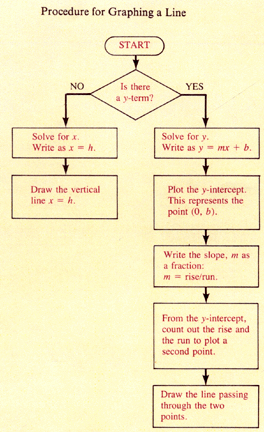# Section 15.1: Cartesian Coordinates and Graphing Lines

## 15.1 Outline

1. Solving equations with two variables
1. satisfy an equation
2. solution
2. Cartesian coordinate system
1. definition
3. coordinates
1. abscissa
2. ordinate
3. Graphing a line by plotting points
1. graph of a line
2. procedure
4. Graphing a line by slope-intercept
1. standard form
2. first-degree equation
3. linear equation
4. independent/dependent variable
5. intercepts
1. y-intercept
2. x-intercept
6. slope
7. slope-intercept form
8. slope point
9. special cases
1. horizontal line
2. vertical line

## 15.1 Essential Ideas

The first-degree equation

y = mx + b

where b is the y-intercept and m is the slope is called the slope-intercept form of the equation of a line.

Procedure for graphing a line: